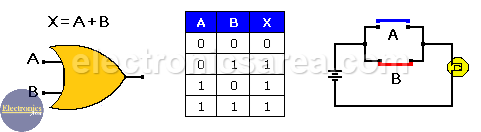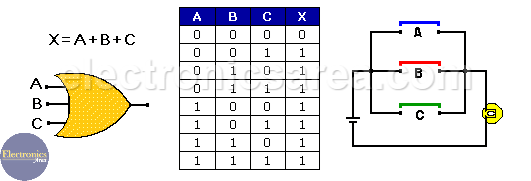# Logic OR gate

The logic Or gate, as the Logic AND gate,  is one of the simplest digital logic gates. The output of this gate is “High”, when one or all of its inputs are “High”. We can also say that, the output of an OR gate is “Low” only when all gate inputs are “Low”.

This gate is represented by the Boolean function: X =A+B for a 2 input OR gate, X = A+B+C for a 3 input gate, X = A+B+C+D for a 4 input gate and so on.

## Two Input OR gate and Truth Table

The following image shows the symbol of a 2 input OR gate and its truth table.

It is also shown how the 2 input OR logic function can be made using switches. By closing the A switch “OR” the B switch, the light will turn ON. “1″= closed, “0”= open,  “0″= light off, “1″= light on.The lamp turns ON when any of the switches (A or B) is closed. That is because, when any of the switches is closed, an electric current flow trough the lamp. The Boolean function is: X = A+B.

An interesting logic gate to see is the XOR gate or EX-OR gate that has a “0” output when the A and B inputs are “1”. Loot at the 2-input OR gate truth table.

## Three Input OR gate and Truth Table

The following picture shows the symbol of a 3 input OR gate and its truth table.

It is also shown how the 3 input OR logic function can be made using switches. By closing the A switch “OR” the B switch “OR” the C switch, the light will turn ON. “1″= closed, “0”= open, “0″= light off, “1″= light on.The lamp turns ON when any of the switches (A or B or C) is closed. That is because, when any of the switches is closed, an electric current flow trough the lamp. The Boolean function is: X = A+B+C.

If we want the opposite behavior, let say that the light bulb goes on when all switches are open, ta NOR gate must be used.

Notes:

• “0”, “Low” and “OFF” have the same meaning.
• “1”, “High” and “ON” have the same meaning.
•
•
•
•
•
•
•
•
•
•
•
•
•
•
•
•
•
•